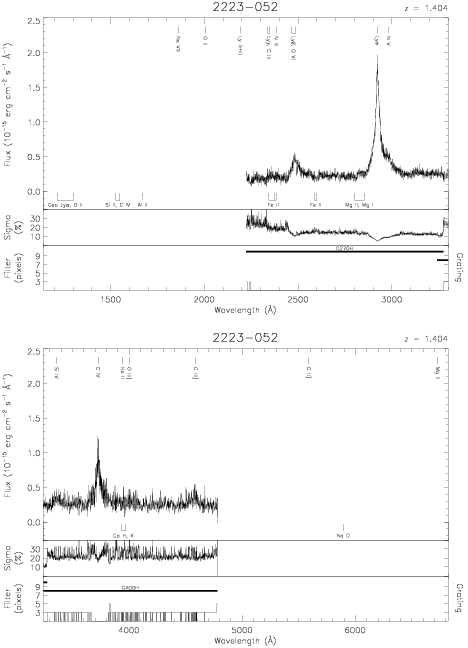Download dataset
TELESCOP= 'HST     '
INSTRUME= 'FOS     '
EQUINOX =               2000.0
DATE-OBS= '1991-09-11T17:59:23'
RA_PNT  =       336.4471250000
DEC_PNT =        -4.9503888889
PA_PNT  =       187.0903000000
OBJECT  = '2223-052'
ROOTNM01= 'Y0PE0M02T'
GRNDMD01= 'SPECTROSCOPY'
DETECT01= 'AMBER   '
APERID01= 'A-1     '
FGWAID01= 'H27     '
ROOTNM02= 'Y0PE0M03T'
GRNDMD02= 'SPECTROSCOPY'
DETECT02= 'AMBER   '
APERID02= 'A-1     '
FGWAID02= 'H27     '
ROOTNM03= 'Y0PE0M04T'
GRNDMD03= 'SPECTROSCOPY'
DETECT03= 'AMBER   '
APERID03= 'A-1     '
FGWAID03= 'H40     '